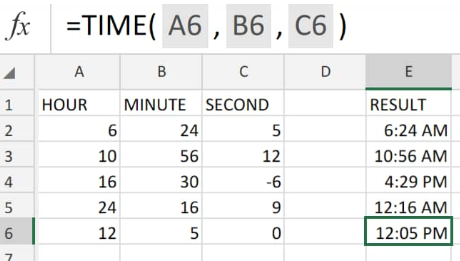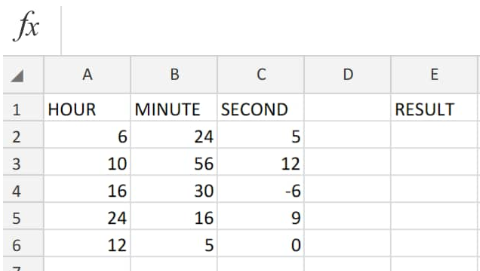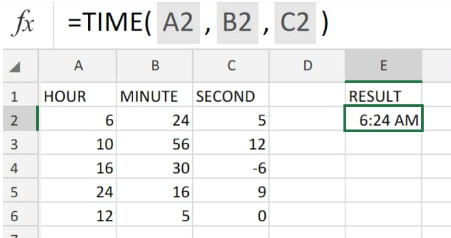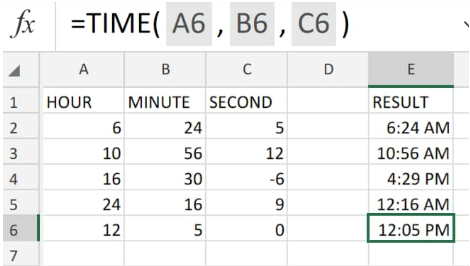Get instant live expert help with Excel or Google Sheets“My Excelchat expert helped me in less than 20 minutes, saving me what would have been 5 hours of work!”

#### Post your problem and you’ll get expert help in seconds.

Your message must be at least 40 characters
Our professional experts are available now. Your privacy is guaranteed.

# How to use Excel time – Excelchat

Excel TIME formulas is an in-built operation which allows us to generate time formats with specified components of hours, minutes, and seconds.

The TIME Excel function is utilized whenever we are required to create a proper time format by using a formula.Figure 1. of Time in Excel

## Generic Formula

`=TIME (hour, minute, second)`

• Hour =. Any duration in Excel between 0 (zero) and 32767 representing hour(s). Any value above 23 is reduced by 24 with the remainder regarded as an hour value;

`TIME(28,0,0) = TIME(4,0,0) = 3:00 AM`

Minute =. Any duration in Excel between 0 and 32767 representing minute(s). Any value above 59 must be converted into hours and minutes;

`TIME(0,720,0) = TIME(12,00,0) = .520803 or 12:00 PM`

• Second =. Any duration in Excel between 0 and 32767 representing second(s). Any value above 59 must be converted into hours, minutes, as well as seconds;

`TIME(0,0,2050) = TIME(0,34,02) = .023148 or 12:34:10 AM`

## How to Use Time Formula in Excel

We will now demonstrate how to create time with hour, minute, and second values by using the Excel formula for time.

1. Enter the specified number values – representing duration in Excel – which we want to use as our time components in labelled columns of our worksheet;Figure 2. of Durations in Excel

Be sure to provide an empty column of cells for the Excel time formulas to return our generated Excel time values (column E above).

1. The Excel time formula we will enter into cell E2 of our worksheet example is as follows;

`=TIME(A2,B2,C2)`Figure 3. of Time in Excel

The formula for time in Excel we entered returned our generated time Excel time value as 6:24 AM; which is based on our specified length of time in Excel.

1. Modify and copy the Excel formulas for time down into the other cells of column E to generate the other time Excel results;Figure 4. of Time in Excel